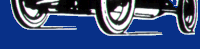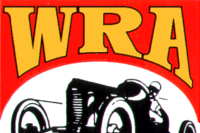HomeAbout WRAHistory of WRAWRA CarsEvent SchedulePhoto GalleryWRA Track InfoReference DeskMembership InfoRelated LinksContact UsClassified AdsWRA MERCHAutomotive Formulas

Formulas for displacement, bore and stroke:

cylinder volume = pi/4 x bore^2 x stroke

stroke = displacement / (pi/4 x bore^2 x number of cylinders)

Formulas for compression ratio:

(CylVolume + ChamberVolume) / ChamberVolume

cylinder volume = pi/4 x bore^2 x stroke

chamber volume = cylinder volume / compression ratio - 1.0

displacement ratio = cylinder volume / chamber volume

amount to mill = (new disp. ratio - old disp. ratio / new disp. ratio x old disp. ratio) x stroke

Formulas for piston speed:

piston speed in fpm = stroke in inches x rpm / 6

rpm = piston speed in fpm x 6 / stroke in inches

Formulas for brake horsepower:

horsepower = rpm x torque / 5252

torque = 5252 x horsepower / rpm

brake specific fuel consumption = fuel pounds per hour / brake horsepower

bhp loss = elevation in feet / 1000 x 0.03 x bhp at sea level

Formulas for indicated horsepower & torque:

horsepower = mep x displcement x rpm / 792,00

torque = mep x displacement / 150.8

mep = hp x 792,000 / displacement x rpm

mep = hp x 792,000 / displacement x rpm

mechanical efficiency = brake output / indocated output x 100

friction output = indicated output - brake output

taxable horsepower = bore2 x cylinders / 2.5

Formulas for air capacity & volumetric efficiency:

theoretical cfm = rpm x displacement / 3456

volumetric efficiency = acutal cfm / theoretical cfm x 100

street carb cfm = rpm x displacement / 3456 x 0.85

racin carb cfm = rpm x displacement / 3456 x 1.1

Formulas for tire size & their effect:

effective ratio = (old tire diameter / new tire diameter) x original ratio

actual mph = (new tire diameter / old tire diameter) x actual mph

Formulas for g force & weight transfer:

drive wheel torque = flywheel torque x first gear x final drive x 0.85

wheel thrust = drive wheel torque / rolling radius

g = wheel thrust / weight

weight transfer = weight x cg height / wheelbase x g

lateral acceleration = 1.227 x raduis / time^2

lateral weight transfer = weight x cg height / wheel track x g

centrufugal force = weight x g

Formulas for shift points:

rpm after shift = ratio shift into / ratio shift from x rpm before shift

drivehsaft torque = flywheel torque x transmission ratio

Formula for instrument error:

actual mph = 3600 / seconds per mile

speedo error percent = difference between actual and indicated speed / actual speed x 100

indicated distance = odometer reading at finish - odometer reading at start

odo error percent = difference between actual and indicated distances / actual distance x 100

Formulas for MPH RPM gears & tires:

mph = (rpm x tire diameter) / (gear ratio x 336)

rpm = (mph x gear ratio x 336) / tire daimeter

gear ratio = (rpm x tire diameter) / (mph x 336)

tire diameter = (mph x gear ratio x 336) / rpm

Formulas for weight distribution:

percent of weight on wheels = weight on wheels / overweight x 100

increased weight on wheels = [ distance of cg from wheels / wheelbase x weight ] + weight

Formulas for center of gravity:

cj location behind front wheels = rear wheel weights / overall weight x wheelbase

cg location off-center to heavy side = track / 2 - [ weight on light side / overall weight ] x track

cg height = [ level wheelbase x raised wheelbase x added weight on scale / distance raised ] x overall weight

WESTERN RACING ASSOCIATION
Celebrating Vintage Racing since 1982